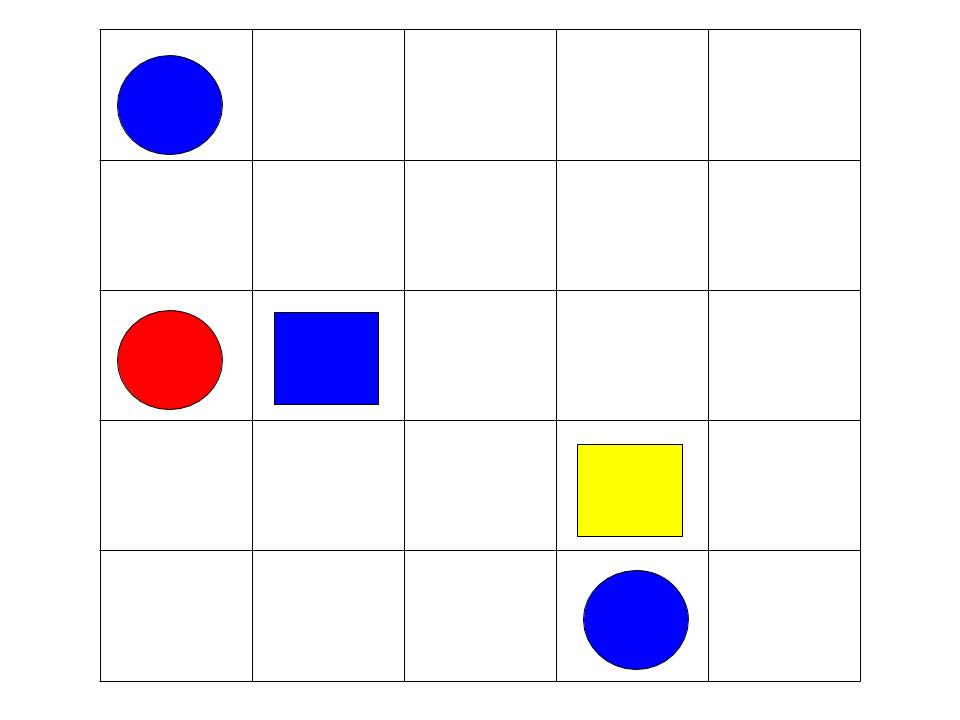# MUN Computer Science 1002 - Lab 3 Predicates and quantifiers

### Materials:

Please bring scratch paper and pens/pencils.
During the quiz, you can use only the paper notes you made solving the lab, as well as the text of the lab itself.

### Review:

• Lectures 9-11.

### Vocabulary

• Canonical CNF, canonical DNF, Boolean functions
• Sets, $\emptyset, \mathbb{N, Z, Q, R}$.
• Predicates, domain (universe) of a predicate, existential and universal quantifiers, predicate logic
• Free and bound variables, scope of quantifiers, prenex form.

## Individual work

Solve each of the following exercises.

1. For each of the following Boolean functions, construct a canonical CNF and a canonical DNF. Hint: you can write the whole truth table, but you don't necessarily need to.
1. $AllTheSame(x,y,z)$ which returns true when either all its inputs are true, or all its inputs are false.
2. $Parity(x,y,z)$ which returns true when an odd number of its inputs are true (that is, either one or three)
2. Let $x = y, x < y, Even(x)$ and $Prime(x)$ be predicates. Translate the following from English to logic (you can use standard arithmetic operations). Make sure to specify domains of quantifiers using $\mathbb{N, Z, Q, R}$, and note which of these statements are true, and which are false.
1. Every integer greater than n is not prime.
2. There is a smallest natural number.
3. If an integer gives the same value when multiplied by two different integers, that integer must be 0. (Hint: in order to ensure that two variables take different values state explicitly that they are not equal.)
3. Which of the following pairs consist of equivalent formulas? That is, when is $F \equiv G$ for formulas below?
1. $F=\forall x \forall y P(x,y)$, $G= \forall y \forall x P(x,y)$
2. $F = \forall x \exists y P(x,y)$, $G = \forall y \exists x P(x,y)$
3. $F=\forall x \forall y P(y,x)$, $G= \forall x \forall y P(x,y)$
4. $F = \forall x \exists y P(x,y)$, $G = \exists x \forall y P(x,y)$
4. Recall that a formula is in prenex form when each quantified variable has a unique name, and all quantifiers are in front of the formula. Now, convert each of the following into prenex form. For simplicity, start by opening up implications using $F \to G \equiv \neg F \vee G$ rule.
1. $\forall x (\exists y P(x,y)) \to (\exists y Q(x,y))$
2. $(( \exists x P(x)) \to (\exists x \neg P(x))) \to (\exists x \forall y P(x) \vee \neg P(y))$
5. For each of the following formulas, negate them and simplify until all negations are on predicates. Use DeMorgan, double negation, definitions of implication and quantifiers.
1. $\forall x \forall y \exists z, P(x,z) \wedge P(y,z) \to Q(x,y)$
2. $\exists x \forall y Q(y,z) \wedge ((\forall z P(x,y,z)) \to Q(x,y))$

## Group exercisesFor this part of the lab, you will be exploring quantified statements in our version of Tarski World, again in groups of three. There, you have a board with pieces of different shapes and colours; these pieces form the universe of Tarski world. For each shape and each colour of a piece, you have a predicate, as well as a few predicates descibing a relation between pieces. In our lab, we will have predicates $Square(x)$, $Circle(x)$, $Red(x), Orange(x), Yellow(x), Green(x), Blue(x),$ as well as $NextTo(x,y)$, which is true iff $y$ is in a cell adjacent to $x$ (left/right/front/back of $x$), and $Aligned(x,y)$, which is true if $x$ and $y$ are either in the same column or in the same row. (Of course, you can use these predicates with any variable names, not just $x$ or $y$).

Now, you can write formulas such as $\exists x Blue(x)$ or $\forall x Square(x) \to \exists y Circle(y) \wedge NextTo(x,y)$, which says that every square has a circle next to it; note that the board on the picture satisfies both these formulas. For conciseness, we will often use just the first letter of the predicate (so the formulas above would be $\exists x B(x)$ and $\forall x (S(x) \to \exists y C(y) \wedge N(x,y))$

For the following exercises, each of you can make one board in exercise 1, write a formula for another's board in exercise 2, and a formula for third person's board in exercise 3; just make sure you all have different boards and formulas. Work together on all of these exercises.

#### Exercise 1:

Make three more boards satisfying $\forall x S(x) \to \exists y C(y) \wedge N(x,y)$ ). Try to use different numbers of pieces, and place them differently on the board.

#### Exercise 2:

For each of these boards, write a formula which is true for that board, but not for the original board. Use quantifiers in all of your formulas.

#### Exercise 3:

Now, for each of your three boards, write a formula which is false for that board, but would become true if you add, remove or move one piece. State which piece needs to be added/removed/moved and where.

## Review after the group exercises

Consider a formula $\exists x Blue(x) \wedge ((\forall y Green(y) \to Aligned(x,y)) \vee (\exists z Square(z) \wedge NextTo(x,z))$

1. Make a board where this formula is true.
2. Make a second board where this formula is false, but changing one piece makes it true.
3. For your second board, write a formula which is true for that board.